# Testing for lack of fit (Reading: Faraway(2005, 1st edition), 6.3)

Variance known

• Using the same data as in the weighted least squares lab, we are going to test whether the model fitted in the previous lab is adequate.

• Because of the way the weights are defined, i.e., wi=1/var(yi), the known variance is implicitly equal to one (why?).

Let's first fit the weighted least square model.

> p # take a look of the data

momentum energy crossx sd

1         4  0.345    367 17

2         6  0.287    311  9

3         8  0.251    295  9

...deleted...

10      150  0.060    192  5

> x <- p[,2]; y <- p[,3]; w <- 1/p[,4]^2 # extract variables and calculate weights

> g <- lm(y~x,weights=w) # fit a simple regression model with w as weights
> summary(g) # take a look of the fitted model

Call:

lm(formula = y ~ x, weights = w)

Residuals:

Min         1Q     Median         3Q        Max

-2.323e+00 -8.842e-01  1.266e-06  1.390e+00  2.335e+00

Coefficients:

Estimate Std. Error t value Pr(>|t|)

(Intercept)  148.473      8.079   18.38 7.91e-08 ***

x            530.835     47.550   11.16 3.71e-06 ***

---

Signif. codes:  0 '***' 0.001 '**' 0.01 '*' 0.05 '.' 0.1 ' ' 1

Residual standard error: 1.657 on 8 degrees of freedom

Multiple R-Squared: 0.9397,     Adjusted R-squared: 0.9321

F-statistic: 124.6 on 1 and 8 DF,  p-value: 3.710e-06

• Look at the high R2 and the very small p-value for overall F-test

• Q: do you think the model is a good fit?

• Now plot the data and the fitted regression line:
> par(mfrow=c(1,2)) # split the graphic window into two

> plot(x, y, cex=2) # draw the scatter plot of energy and crossx
> abline(g\$coef) # add the fitted line

Now, let's also plot the residuals against crossx.

> plot(x, g\$res, cex=2); abline(0, 0) # draw scatter plot of residuals and crossx and add y=0 line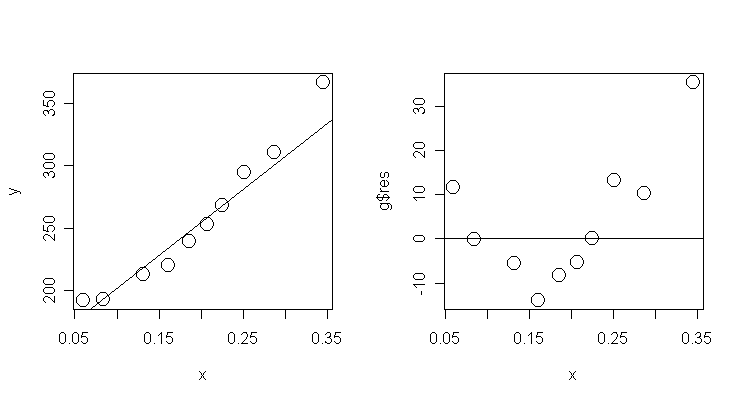• Q: What have you found from the plots?

• Q: Whether or not the plots show that the fitted model is not good enough?

• Let's perform the test for lack of fit. Compute the test statistic and its p-value:

> (8*1.657^2)/1 # test statistics = (n-p)*σ-hat2/σ2

 21.96519

> 1-pchisq(21.96519, 8) # p-value of the test, the "pchisq" command returns the cdf value of a chi-square distribution

 0.004980762

• Because the p-value is small, we conclude that there is a lack of fit.

• Notice that although the original linear model had an R2 of 94% and a very small overall F p-value, this in itself is not enough to conclude that this particular model fits.

Let us try to add a quadratic term, as suggested by the residual plot above, to the model and test again:

> g1 <- lm(y ~ x + I(x^2), weights=w) # fit a 2nd-order polynomial model
> summary(g1, cor=T) # take a look of the new fitted model

Call:

lm(formula = y ~ x + I(x^2), weights = w)

Residuals:

Min       1Q   Median       3Q      Max

-0.89928 -0.43508  0.01374  0.37999  1.14238

Coefficients:

Estimate Std. Error t value Pr(>|t|)

(Intercept)  183.8305     6.4591  28.461  1.7e-08 ***

x              0.9709    85.3688   0.011 0.991243

I(x^2)      1597.5047   250.5869   6.375 0.000376 ***

---

Signif. codes:  0 '***' 0.001 '**' 0.01 '*' 0.05 '.' 0.1 ' ' 1

Residual standard error: 0.6788 on 7 degrees of freedom

Multiple R-Squared: 0.9911,     Adjusted R-squared: 0.9886

F-statistic: 391.4 on 2 and 7 DF,  p-value: 6.554e-08

Correlation of Coefficients:

(Intercept) x

x      -0.94

I(x^2)  0.86       -0.97

• The quadratic term is significant.

• The linear term becomes insignificant because of the strong collinearity between linear and quadratic terms.

• However, it doesn't mean we should just drop the linear term. A better solution is to rescale the linear and quadratic terms so that they can be (almost) orthogonal.

• Let's perform the test for lack of fit on the new model.

> (7*0.6788^2)/1  # calculate test statistic

 3.225386

> 1-pchisq(3.225386, 7) # calculate p-value

 0.8633989

• This time we cannot detect a lack of fit.

• Let's plot the fits of the two models and a residual plot under the new model:

> plot(x, y, cex=2) # draw the scatter plot of energy and crossx
> abline(g\$coef) # add the fitted line of model g

> xm <- seq(0.05, 0.35, 0.01); lines(xm, g1\$coef+g1\$coef*xm+g1\$coef*xm^2, lty=2) # add the fitted line of model g1

> plot(x, g1\$res, cex=2); abline(0, 0) # a residual plot under model g1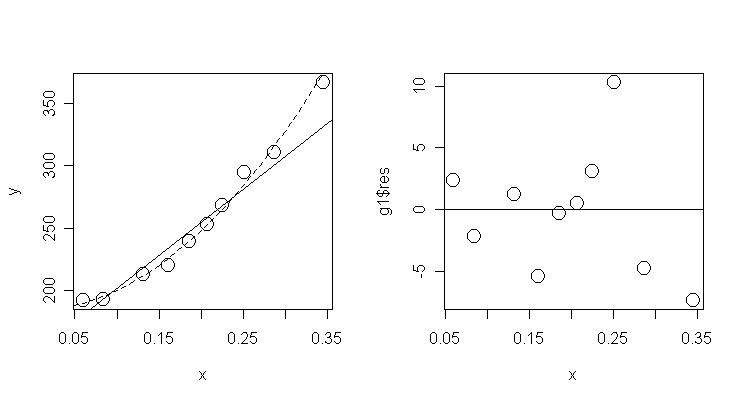• The quadratic model seems more appropriate than the linear model.

Variance unknown

• The data for this example consist of thirteen specimens of 90/10 Cu-Ni alloys with varying iron content in percent.

• The specimens were submerged in sear water for 60 days and the weight loss due to corrosion was recorded in units of milligrams per square decimeter per day.

> corrosion # take a look of the data

Fe  loss

1  0.01 127.6

2  0.48 124.0

3  0.71 110.8

4  0.95 103.9

5  1.19 101.5

6  0.01 130.1

7  0.48 122.0

8  1.44  92.3

9  0.71 113.1

10 1.96  83.7

11 0.01 128.0

12 1.44  91.4

13 1.96  86.2

• Notice that this is a data with some replication.

• Q: What is the source of variation in the replication?

> g <- lm(loss ~ Fe, data=corrosion) # Fit a simple linear model with loss as response and Fe as predictor
> summary(g) # take a look of the fitted model

Call:

lm(formula = loss ~ Fe, data = corrosion)

Residuals:

Min      1Q  Median      3Q     Max

-3.7980 -1.9464  0.2971  0.9924  5.7429

Coefficients:

Estimate Std. Error t value Pr(>|t|)

(Intercept)  129.787      1.403   92.52  < 2e-16 ***

Fe           -24.020      1.280  -18.77 1.06e-09 ***

---

Signif. codes:  0 '***' 0.001 '**' 0.01 '*' 0.05 '.' 0.1 ' ' 1

Residual standard error: 3.058 on 11 degrees of freedom

Multiple R-Squared: 0.9697,     Adjusted R-squared: 0.967

F-statistic: 352.3 on 1 and 11 DF,  p-value: 1.055e-09

• Look at the high R2 and the very small p-value for overall F-test.

• Q: Do you think this is a good fit?

• Let's check the fit graphically:

> plot(corrosion\$Fe, corrosion\$loss, ylim=c(80,140), cex=2) # draw the scatter plot of Fe and loss
> abline(g\$coef) # add the fitted line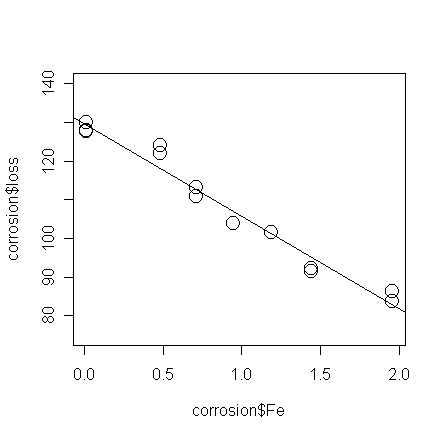• Examine the plot and guess whether the test for lack of fit will be accepted or rejected.

To perform the test for lack of fit,

• We now fit a saturated model that reserves a parameter for each group of data with the same value of Fe.

• This is accomplished by declaring the predictor to be a factor in R.

> ga <- lm(loss ~ factor(Fe), data=corrosion) # fit the saturated model, the "factor" command treats Fe as a qualitative predictor

> summary(ga) # take a look of the fitted saturated model

Call:

lm(formula = loss ~ factor(Fe), data = corrosion)

Residuals:

Min         1Q     Median         3Q        Max

-1.250e+00 -9.667e-01  9.991e-17  1.000e+00  1.533e+00

Coefficients:

Estimate Std. Error t value Pr(>|t|)

(Intercept)     128.567      0.809 158.914 4.19e-12 ***

factor(Fe)0.48   -5.567      1.279  -4.352  0.00481 **

factor(Fe)0.71  -16.617      1.279 -12.990 1.28e-05 ***

factor(Fe)0.95  -24.667      1.618 -15.245 5.03e-06 ***

factor(Fe)1.19  -27.067      1.618 -16.728 2.91e-06 ***

factor(Fe)1.44  -36.717      1.279 -28.703 1.18e-07 ***

factor(Fe)1.96  -43.617      1.279 -34.097 4.24e-08 ***

---

Signif. codes:  0 '***' 0.001 '**' 0.01 '*' 0.05 '.' 0.1 ' ' 1

Residual standard error: 1.401 on 6 degrees of freedom

Multiple R-Squared: 0.9965,     Adjusted R-squared: 0.9931

F-statistic: 287.3 on 6 and 6 DF,  p-value: 4.152e-07

Notice that

• the saturated model has 7 parameters (including a constant term), which is equal to the number of distinct values of Fe.

• The residual standard error, 1.401, is a pure error estimate of true σ.

• The fitted values of the saturated model are the means in each group - put these on the plot:

> points(corrosion\$Fe, ga\$fit, pch=18, cex=2) # add the means in each group to the plot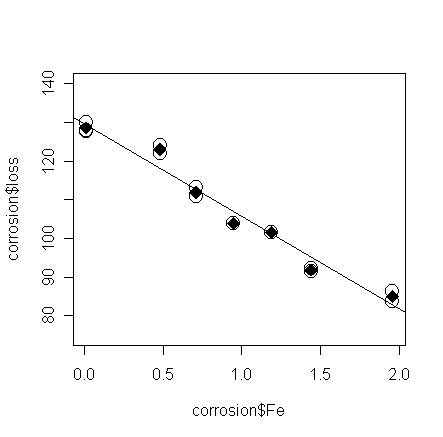• From the plot, do you think the model can pass the test for lack of fit?

• Here, let's calculate the SS and degrees of freedom for the pure error "by hand":

> sum(ga\$res^2) # calculate SSp.e.

 11.78167

> ga\$df # the dfp.e.

 6

> sum(g\$res^2) # the RSS calculated from the model g

 102.8502

We can put these values into the ANOVA table to calculate test statistic:

 d.f. SS MS F Residual n-p  (11) RSS  (102.8502) Lack of fit n-p-dfp.e. (5) RSS-SSp.e. (RSS-SSp.e.)/(n-p-dfp.e. ) ratio of MS Pure error dfp.e.  (6) SSp.e.  (11.78167) (SSp.e.)/(dfp.e.)

And, now the test statistic:

> ((102.8502-11.78167)/(11-6))/((11.78167/6)) # test statistic for lack of fit

 9.275615

> 1-pf(9.275615, 5, 6) # the p-value

 0.008622849

• A slightly quicker way to get the same result:

> anova(g, ga) # compute the ANOVA table for models g and ga

Analysis of Variance Table

Model 1: loss ~ Fe

Model 2: loss ~ factor(Fe)

Res.Df     RSS Df Sum of Sq      F   Pr(>F)

1     11 102.850

2      6  11.782  5    91.069 9.2756 0.008623 **

---

Signif. codes:  0 '***' 0.001 '**' 0.01 '*' 0.05 '.' 0.1 ' ' 1

• The low p-value indicates that we must conclude that there is a lack of fit.

Let's try to add more higher order polynomial terms one-by-one until the model pass the test for lack of fit:

> g1 <- lm(loss~Fe+I(Fe^2), data=corrosion) # fit a 2nd-order polynomial model
> anova(g1, ga)
# compute the ANOVA table for models g1 and ga

Analysis of Variance Table

Model 1: loss ~ Fe + I(Fe^2)

Model 2: loss ~ factor(Fe)

Res.Df     RSS Df Sum of Sq      F   Pr(>F)

1     10 100.086

2      6  11.782  4    88.305 11.243 0.005949 **

---

Signif. codes:  0 '***' 0.001 '**' 0.01 '*' 0.05 '.' 0.1 ' ' 1

> g2 <- lm(loss~Fe+I(Fe^2)+I(Fe^3), data=corrosion) # fit a 3rd-order polynomial model
> anova(g2, ga)
# compute the ANOVA table for models g2 and ga

Analysis of Variance Table

Model 1: loss ~ Fe + I(Fe^2) + I(Fe^3)

Model 2: loss ~ factor(Fe)

Res.Df    RSS Df Sum of Sq      F  Pr(>F)

1      9 45.051

2      6 11.782  3    33.269 5.6476 0.03506 *

---

Signif. codes:  0 '***' 0.001 '**' 0.01 '*' 0.05 '.' 0.1 ' ' 1

> g3 <- lm(loss~Fe+I(Fe^2)+I(Fe^3)+I(Fe^4), data=corrosion) # fit a 4th-order polynomial model
> anova(g3, ga)
# compute the ANOVA table for models g3 and ga

Analysis of Variance Table

Model 1: loss ~ Fe + I(Fe^2) + I(Fe^3) + I(Fe^4)

Model 2: loss ~ factor(Fe)

Res.Df    RSS Df Sum of Sq      F  Pr(>F)

1      8 34.975

2      6 11.782  2    23.194 5.9059 0.03822 *

---

Signif. codes:  0 '***' 0.001 '**' 0.01 '*' 0.05 '.' 0.1 ' ' 1

> g4 <- lm(loss~Fe+I(Fe^2)+I(Fe^3)+I(Fe^4)+I(Fe^5), data=corrosion) # fit a 5th-order polynomial model
> anova(g4, ga)
# compute the ANOVA table for models g4 and ga

Analysis of Variance Table

Model 1: loss ~ Fe + I(Fe^2) + I(Fe^3) + I(Fe^4) + I(Fe^5)

Model 2: loss ~ factor(Fe)

Res.Df    RSS Df Sum of Sq      F Pr(>F)

1      7 14.465

2      6 11.782  1     2.683 1.3663 0.2868

Finally, the 5th-order polynomial model passes the test for lack of fit.

• This model is very close to the saturated model (the 6th-order polynomial model is a saturated model, why? Hint. count the number of parameters).

• Let's plot the fitted line of the 5-th order polynomial model and compare it with the straight line model:

> grid <- seq(0, 2, length=50); lines(grid, predict(g4,data.frame(Fe=grid)), lty=2) # add the fitted line of the 5-th order model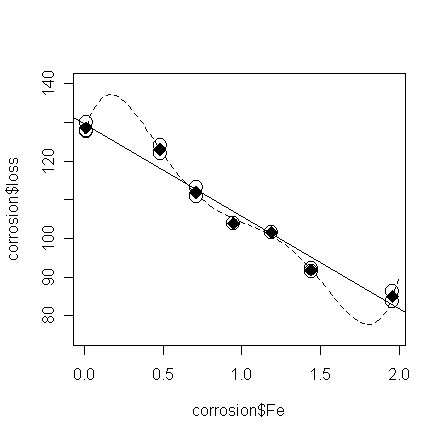• Do you think the 5th order polynomial model is better than the straight line model?

• We actually cannot explain why the corrosion loss should increase then decrease in the region 0<Fe<0.5, and decrease then increase in the region 1.5<Fe<2.0.

• This is probably a consequence of over-fitting the data.

• Because of the complexity of the model, the fitting on the observed Fe's is good, but the prediction on the region without observed Fe's becomes unstable.

• In this case, we may want to stay with the straight line model and consider other reasons that may causes the lack of fit.

• For example, you may want to find out whether the replicates are genuine.

• Perhaps, the low pure error estimate can be explained by some correlation in the measurements.

• The source of variation should also be examined.

• For example, if the specimens with same Fe's are taken from different positions of a same unit, the pure error estimate does not include the variation between different units.

• In the case, it is reasonable to get a pure error estimate lower than expected.

• Other possible explanation is unmeasured predictors is causing the lack of fit.